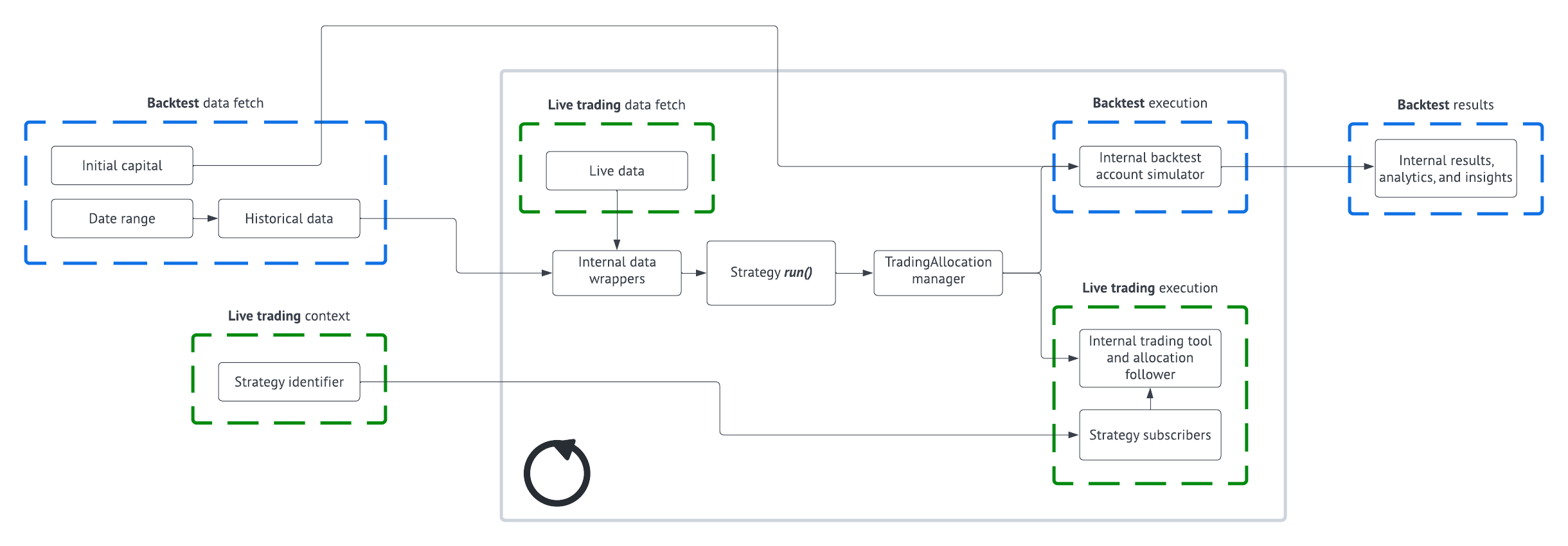# Strategy Builder Overview

## System Overview## Writing a Strategy

When writing a strategy, the first step is to import the Strategy and TargetAllocation classes like so:

``````from surmount.base_class import Strategy, TargetAllocation
``````

Next, import the technical indicators and data sources that you want to use for your strategy. For more information, scroll down to the example strategies or click on the links below:

After that, define your strategy class, interval, assets, and data. The interval can be one of the following: `1min`, `5min`, `1hour`, `4hour`, `1day`. The data list should be a list of the data classes you imported earlier.

``````class TradingStrategy(Strategy):

def __init__(self):
self.tickers = ["AAPL", ...]
self.data_list = [...]

@property
def interval(self):
return "1day"

@property
def assets(self):
return self.tickers

@property
def data(self):
return self.data_list
``````

Finally, define your strategy in the `run()` method. The `data` object is a Python dictionary containing OHLCV data as well as data from all of the sources you added to data_list above. This method needs to return a TargetAllocation object, which is a dictionary with the keys being tickers and values being decimal allocations that add up to 1 at the most.

``````def run(self, data):
allocation_dict = {i: 1/len(self.tickers) for i in self.tickers}
# WRITE YOUR STRATEGY LOGIC HERE
return TargetAllocation(allocation_dict)
``````

## Example Strategies

Here is a simple strategy that checks the latest insider trade for sales:

``````from surmount.base_class import Strategy, TargetAllocation
from surmount.technical_indicators import RSI, EMA, SMA, MACD, MFI, BB
from surmount.logging import log
from surmount.data import Asset, InstitutionalOwnership, InsiderTrading

def __init__(self):
self.tickers = ["SPY", "QQQ", "AAPL", "GOOGL"]
self.data_list = [InstitutionalOwnership(i) for i in self.tickers]
self.data_list += [InsiderTrading(i) for i in self.tickers]

@property
def interval(self):
return "1day"

@property
def assets(self):
return self.tickers

@property
def data(self):
return self.data_list

def run(self, data):
allocation_dict = {i: 1/len(self.tickers) for i in self.tickers}
for i in self.data_list:
if data[tuple(i)] and len(data[tuple(i)])>0:
if "Sale" in data[tuple(i)][-1]['transactionType']:
allocation_dict[tuple(i)] = 0

return TargetAllocation(allocation_dict)
``````

This is an interesting strategy that buys QQQ midday if there is a V shape in the last 3 candles:

``````from surmount.base_class import Strategy, TargetAllocation
from surmount.technical_indicators import RSI, EMA, SMA, MACD, MFI, BB
from surmount.logging import log

@property
def assets(self):
return ["QQQ"]

@property
def interval(self):
return "1hour"

def run(self, data):
d = data["ohlcv"]
qqq_stake = 0
if len(d)>3 and "13:00" in d[-1]["QQQ"]["date"]:
v_shape = d[-2]["QQQ"]["close"]<d[-3]["QQQ"]["close"] and d[-1]["QQQ"]["close"]>d[-2]["QQQ"]["close"]
log(str(v_shape))
if v_shape:
qqq_stake = 1

return TargetAllocation({"QQQ": qqq_stake})
``````

This is a strategy that buys \$VIRT when there is a spike in SPY trading volume:

``````from surmount.base_class import Strategy, TargetAllocation
from surmount.logging import log
from surmount.data import Asset, InstitutionalOwnership
import pandas_ta as ta
import pandas as pd

def SMAVol(ticker, data, length):
'''Calculate the moving average of trading volume

:param ticker: a string ticker
:param data: data as provided from the OHLCV data function
:param length: the window

:return: list with float SMA
'''
close = [i[ticker]["volume"] for i in data]
d = ta.sma(pd.Series(close), length=length)
if d is None:
return None
return d.tolist()

def __init__(self):
self.tickers = ["VIRT"]
self.data_list = []

@property
def interval(self):
return "1day"

@property
def assets(self):
return self.tickers

@property
def data(self):
return self.data_list

def run(self, data):
vols = [i["VIRT"]["volume"] for i in data["ohlcv"]]
smavols = SMAVol("VIRT", data["ohlcv"], 40)
smavols2 = SMAVol("VIRT", data["ohlcv"], 10)

if len(vols)==0:
return TargetAllocation({})

if smavols2[-1]/smavols[-1]-1>0:
out = smavols2[-1]/smavols[-1]-1
else: out = 0

return TargetAllocation({"VIRT": min(0.9, (out*10)**(0.5))})
``````

This strategy uses pyramiding and Bollinger bands to build long and short positions on \$QQQ.:

``````from surmount.base_class import Strategy, TargetAllocation
from surmount.technical_indicators import SMA, BB
from surmount.logging import log

@property
def assets(self):
return ["QQQ", "SQQQ"]

@property
def interval(self):
return "1hour"

def run(self, data):
holdings = data["holdings"]
data = data["ohlcv"]

sqqq_stake = 0
qqq_stake = 0

qqq_bbands = BB("QQQ", data, 20, 1.4)
qqq_ma = SMA("QQQ", data, 5)

if len(data)<20:
return TargetAllocation({})

current_price = data[-1]["QQQ"]['close']

if qqq_bbands is not None and current_price < qqq_bbands['lower'][-1] and qqq_ma[-1]>qqq_ma[-2]:
log("going long")
if holdings["QQQ"] >= 0:
qqq_stake = min(1, holdings["QQQ"]+0.1)
else:
qqq_stake = 0.4
elif qqq_bbands is not None and current_price > qqq_bbands['upper'][-1]:
log("going short")
if holdings["SQQQ"] >= 0:
sqqq_stake = min(1, holdings["SQQQ"]+0.075)
else:
sqqq_stake = 0.2
else:
log("meh")
qqq_stake = 0
sqqq_stake = 0

return TargetAllocation({"SQQQ": sqqq_stake, "QQQ": qqq_stake})
``````